# Answer to 7.10 => https://www.chegg.com/homework-help/Modeling-and-Analysis-of-Dynamic-Systems...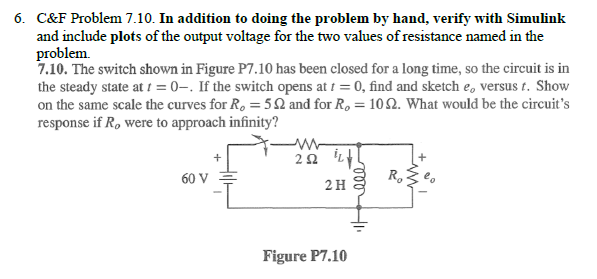6. C&F Problem 7.10. In addition to doing the problem by hand, verify with Simulinlk and include plots of the output voltage for the two values of resistance named in the 7.10. The switch shown in Figure P7.10 has been closed for a long time, so the circuit is in the steady state at t = 0-. If the switch opens at t = 0, find and sketch eo versus t. Show on the same scale the curves for Ro 52 and for Ro 10S2. What would be the circuit's response if Ro were to approach infinity? 60 V R, 2 H Figure P7.10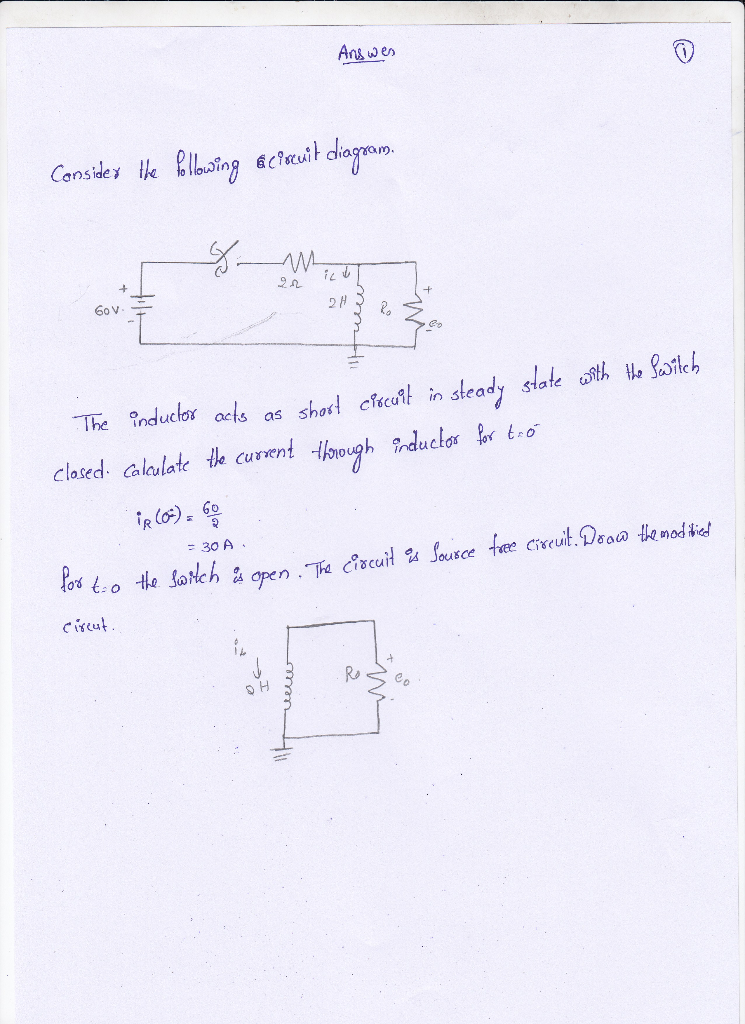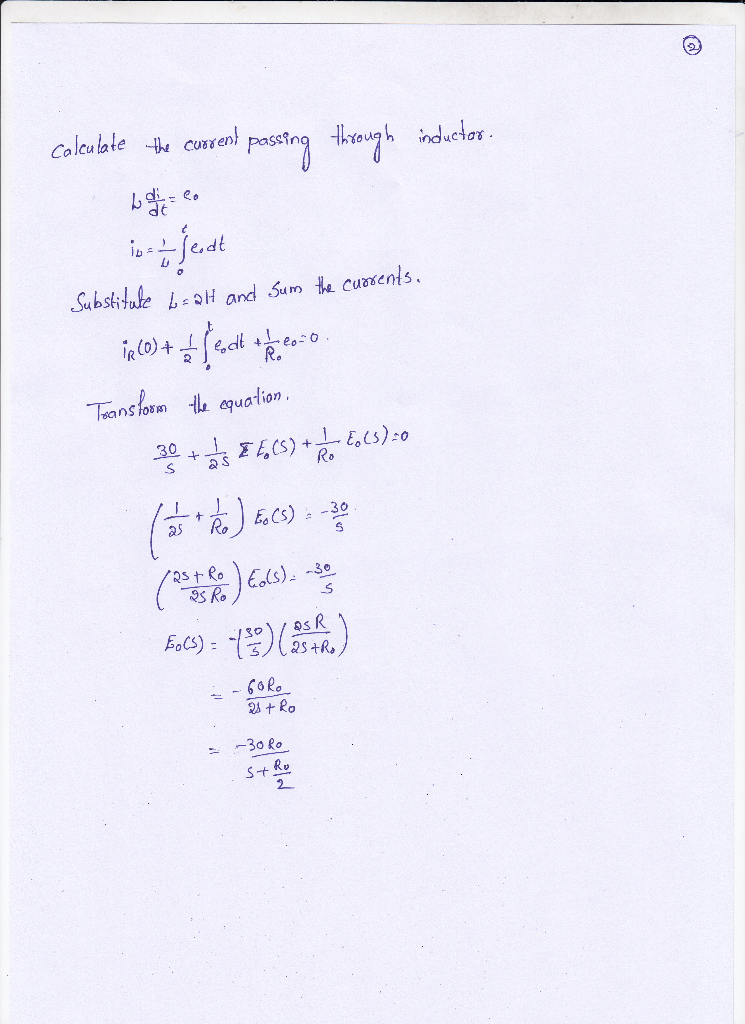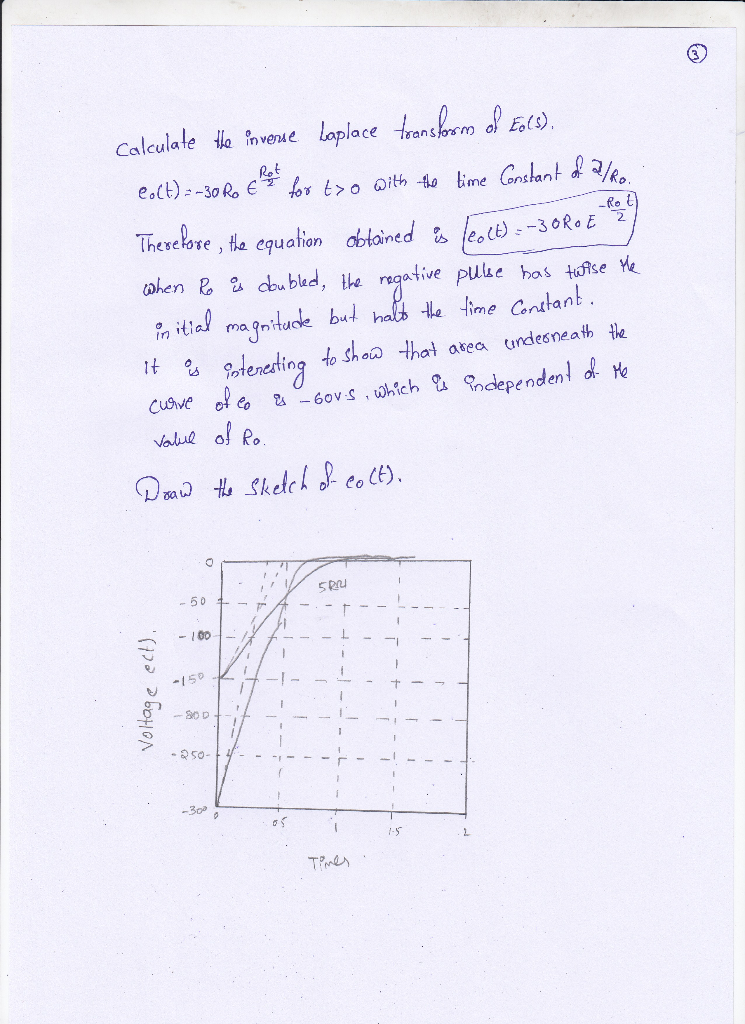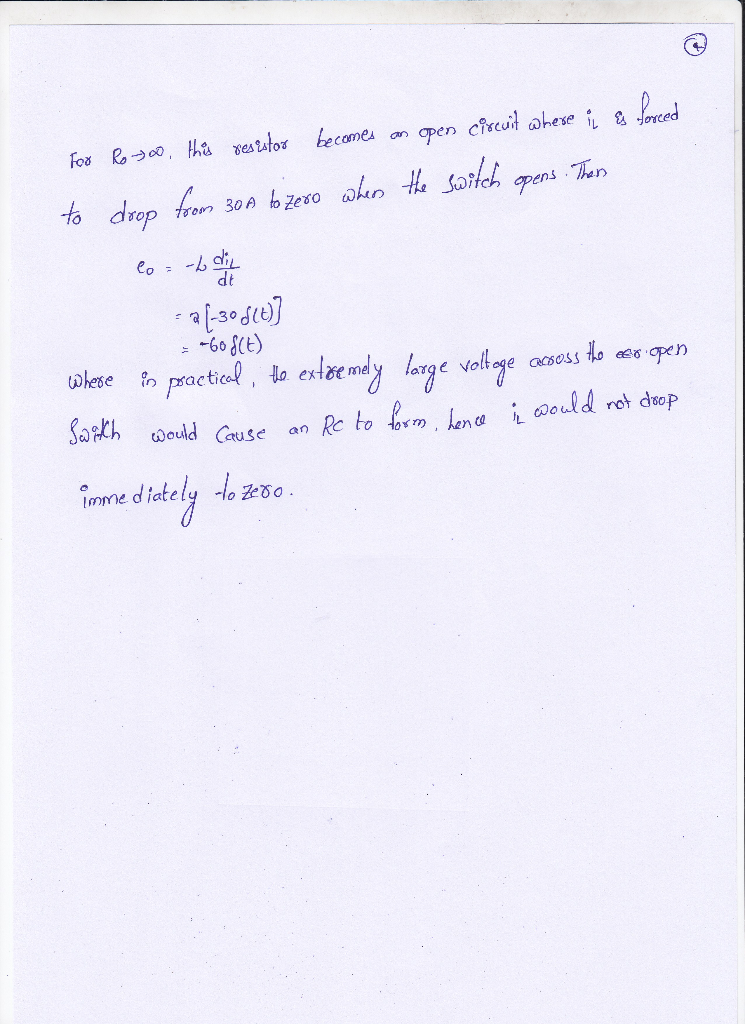More Homework Help Questions Additional questions in this topic.